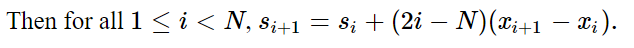# Need help to understand solution to this problem - USACO 2020

Problem link: TRIANGLES
Solution link: SOLUTION
I don’t know how to prove the formulaThe formula should actually be s_i = s_{i-1} + (2i-N)(x_{i+1}-x_i). It helps to think about distances on a number line.

Let d = x_i-x_{i-1}. For 0 \le j < i, |x_i-x_j| = |x_{i-1}-x_j| + d, so we’ll need to add d exactly i times. For i \le j < N, |x_j-x_i| = |x_j - x_{i-1}| - d, so we’ll need to subtract d exactly N-i times. Thus, the net change from s_i to s_{i+1} is d(i-(N-i)) = d(2i-N).

1 Like

The formula is correct, as the x_i are one-indexed.

2 Likes